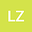Resistance distance-based graph invariants and spanning trees of graphs derived from the strong prism of a graphs with given degree sequence
•• Leilei Zhang
Leilei Zhang
East China Normal University

Corresponding Author:[email protected]

Author Profile## Abstract

Let $G^2_n$ be the graph obtained by the strong prism of a graph $G_n$ with a given degree sequence, i.e. the strong product of $K_2$ and $G_n$. In this paper, we give the relationship between $G_n^2$ and $G_n$ for Kirchhoff index and the total number of spanning tress. which generalized the main results of Z.M. Li et al. ( Appl. Math. Comput., 2020, 382:125335), Y.G. Pan et al. ( Int. J. Quantum Chem. 118 (2018) . E25787) and Y.G. Pan et al. (2019, ArXiv: 1906.04339). We also presented the explicit expressions for the multiplicative degree-Kirchhoff indices of $G_n^2$ when $G_n$ is regular. Typically, Using this results we get the explicit expressions for the Kirchhoff index, multiplicative degree-Kirchhoff and the total number of spanning trees of the strong prism of a wheel $W_n$, respectively. It is surprising to find that the quotient of Kirchhoff (resp. multiplicative degree-Kirchhoff) index of $W_n^2$ and its Wiener (resp. Gutman) index is almost a constant. More specially, let $\mathcal{G}^2_{n,r}$ be the set of subgraphs obtained by randomly deleting $r$ vertical edges from $G^2_n$, where $0\leq r \leq n$. Explicit formulas for Kirchhoff index and number of spanning trees for any graph $G^2_{n,r}\in \mathcal{G}^2_{n,r}$ are established, respectively.